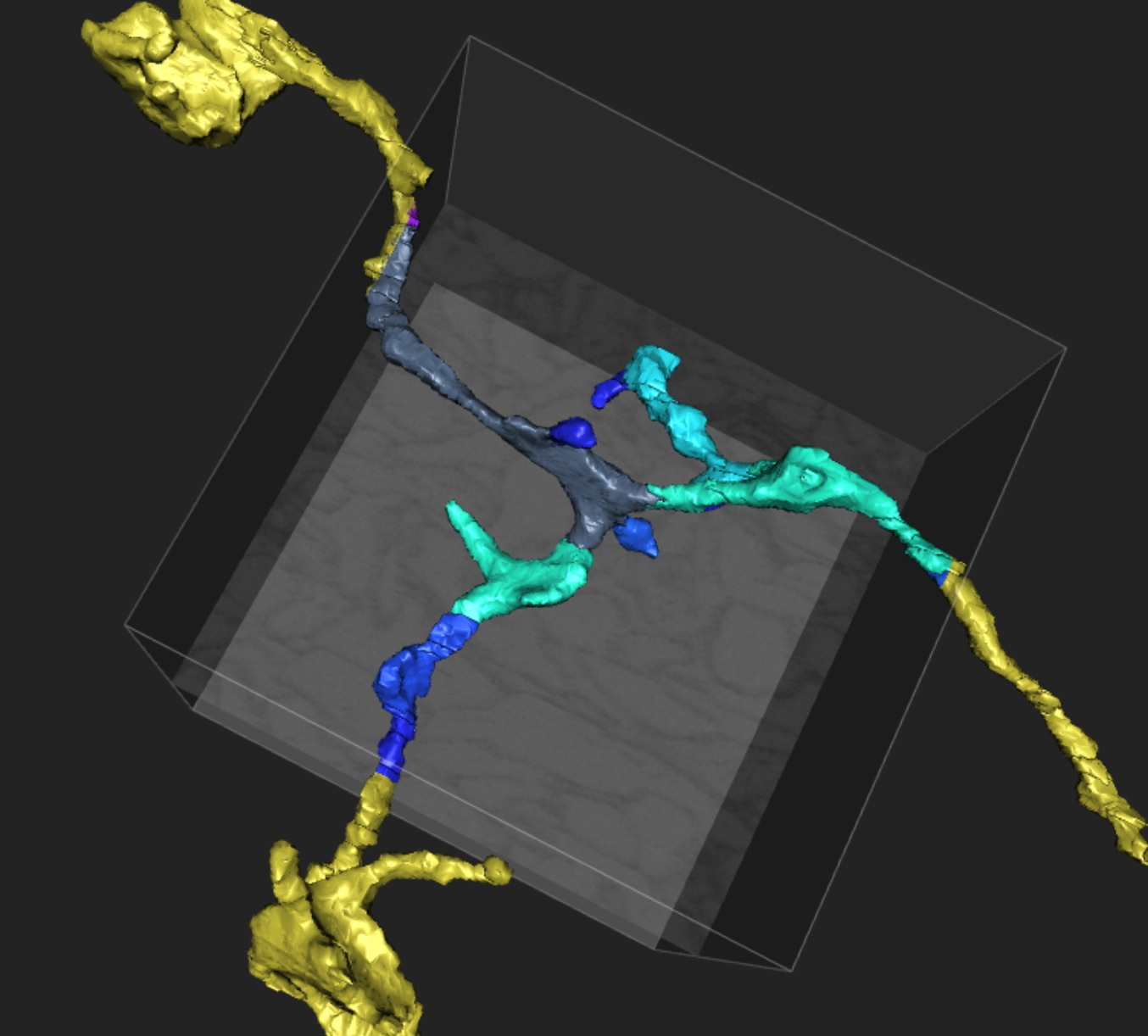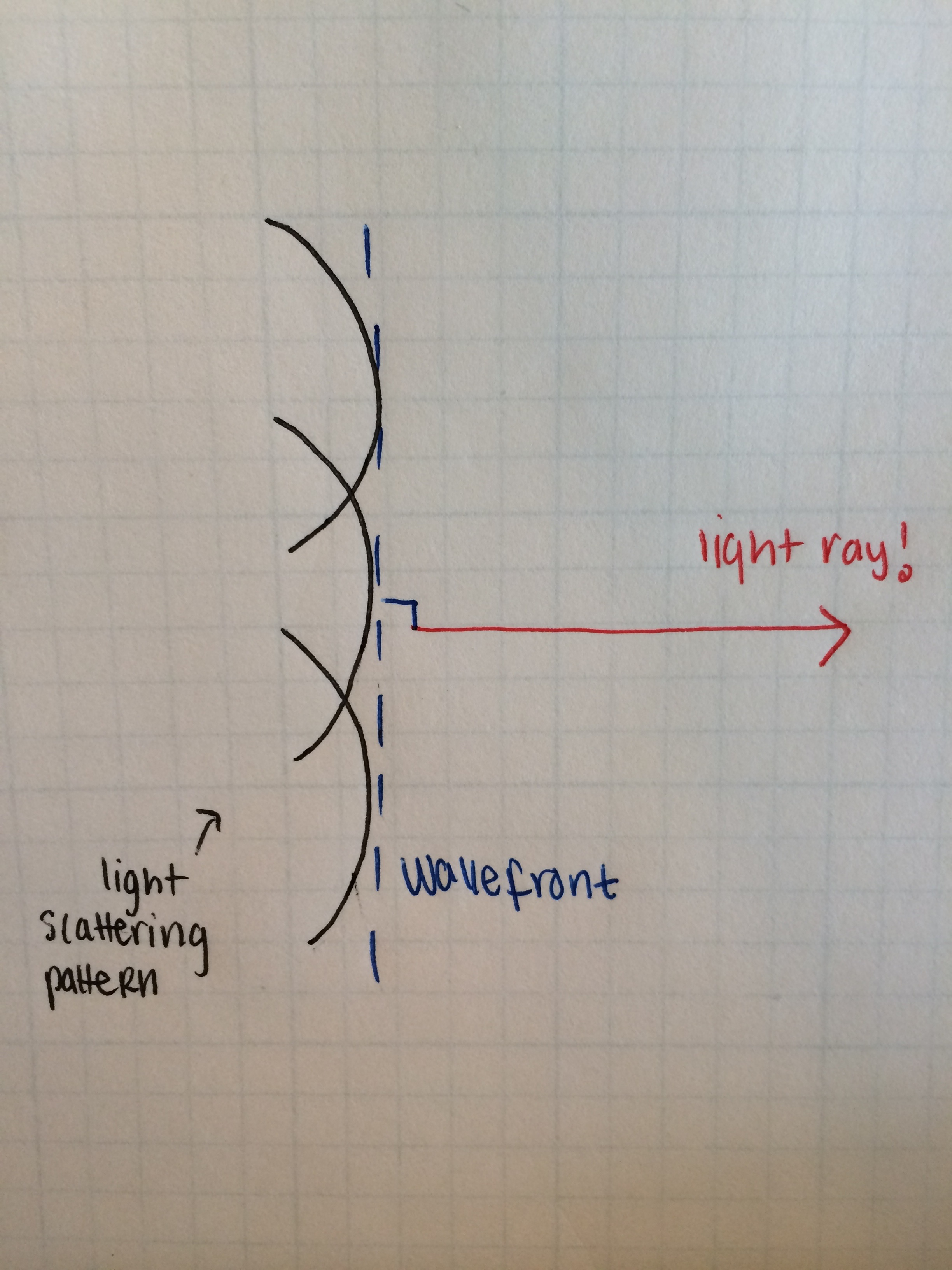# History of Imaging – Part 1The cubes we use in EyeWire come from data collected from a microscope at the Max Planck Institute of Medical Research in Germany – you can read more about the specific microscope that was used here. The microscope is in the family of scanning electron microscopes which are the result of years of research into the nature of light, the study of optics, and the invention of the optical microscope. Observations of optics go back as far as 1200 BCE, and physicist-philosophers like Aristotle, Plato, and Euclid have long speculated about its origins and qualities. Let’s start with the nature of light and follow the evolution of technology and knowledge to end up with the SEMs (Scanning Electron Microscopes) that we now use. This is the story about how we went from macro drawn anatomical diagrams to the beautiful images that we now have of neurons.

Going from this

to this!

The latter image is actually an award winning photo of a hippocampal neuron taken by Dr. Boyle for Nikon’s 2013 Photomicrography Competition.  This once again illustrates just how far we’ve come! Physicists have been studying light for thousands of years and we are now at the point where we can have competitions to photograph tiny entities that highlight beauty.

Part 1: Light and a Quantum of Optics

Light, for a concept that seems so natural to us, has remarkably tricky properties that have shaped the theories of physics* . To start, light is a form of electromagnetic radiation and some aspects of the nature of light can be explained using a set of differential equations known as Maxwell’s equations.The upside down triangle is the Laplacian function, E denotes the electric field, and B denotes the magnetic field. Maxwell’s equations describe the properties of the electric and magnetic fields.

I won’t go through the derivation itself but from these equations one can get to the wave equation. The wave equation describes how a physical wave moves through time and space.  The wave equation that is derived from Maxwell equations, though,  moves at precisely the speed of light, c or 3 x 109 m/s. It looks we derived a wave equation that describes a light wave from electromagnetic theory!

Wave-particle duality is well known phenomena at this point (it’s come up 3 or 4 times during The Big Bang Theory!) We’ve discussed the wave-like nature of light which came from the Maxwell’s equations but now let’s go into the particle nature of light.

When a beam of light is focused on some metals, electrons are emitted from that metal. This is called the photoelectric effect. The effect couldn’t be explained by electromagnetic theory because it occurred at varying intensities of light – the electrons only need to reach a certain frequency in order to be emitted. Einstein theorized that this happened because the electromagnetic field was quantized – that is, instead of waves there were “wave packets” or quanta of energy. These quanta are known as photons. Einstein won a Nobel Prize in 1921 for this observation.
So, should we think of light as a particle or wave? It’s a contentious question that hasn’t yet been answered, and to study it more we would need to go into quantum theory. We don’t need to get into that –  to study optics we want to use light rays which are the “tools” to understand optical phenomena like mirrors. What are light rays? Light scattering in a medium results in the propagation of a wavefront through matter. By defining a line perpendicular to a wave front we can define a highly idealized light ray.There are only two laws that are necessary to understand most of the simple applications of optics; these are the Law of Reflection and Snell’s law. They’re both quite intuitive because we can directly observe the effects.

The law of reflection states that the angle of incidence of the ray of light is equal to the angle of reflection.